Lecture 18: Interfaces

Overview

1. Finishing Up Generics
2. Comparing Items
3. Interfaces

Last Time

1. can be used to store any specified type of object
2. each instance only stores one type
3. Java checks that correct types are used

Generic Syntax

Declare class with a type, T:

public class GenericList<T> {
...
}

Inside the class, T can be used like a variable representing the type:

// add an item of type T
public void add (T item) {
...
}

// get next item of type T
public T next () {
...
}

Better Code with Generics

Now we can use the same GenericList to store any type of object:

GenericList<Animal> zoo = new GenericList<Animal>();
GenericList<Person> school = new GenericList<Person>();
GenericList<Integer> numbers = new GenericList<Integer>();
...

Iterating over the List

We also implemented methods for iteration:

• hasNext() returns true if there is another element in the list
• next() returns the next element in the list

To access all the elements in the list:

while (zoo.hasNext()) {
Animal a = zoo.next();
...
}

public void add (T item) {
Node nd = new Node(item);

if (tail != null) {
tail.setNext(nd);
tail = nd;
} else {
tail = nd;
iter = nd;
}
}

When we call list.add(item), the item is added to the end of the list

The Order of Things

Consider

GenericList<Integer> list = new GenericList<Integer>();

while (list.hasNext()) {
System.out.println(list.next());
}

What does it print?

A Question

Suppose we wanted to store/print elements in sorted order:

SomeList<Integer> list = new SomeList<Integer>();
list.insert(4);
list.insert(2);
list.insert(3);

while (list.hasNext()) {
System.out.println(list.next());
}

We want to see output:

2
3
4

How might we accomplish this?

Sorted List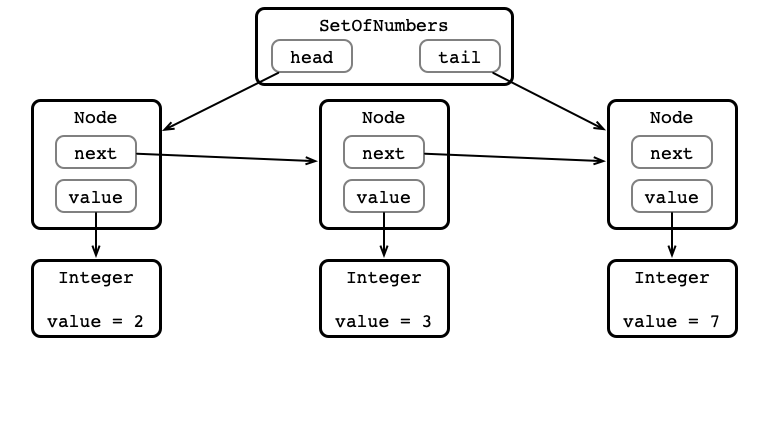How Would We Insert 5?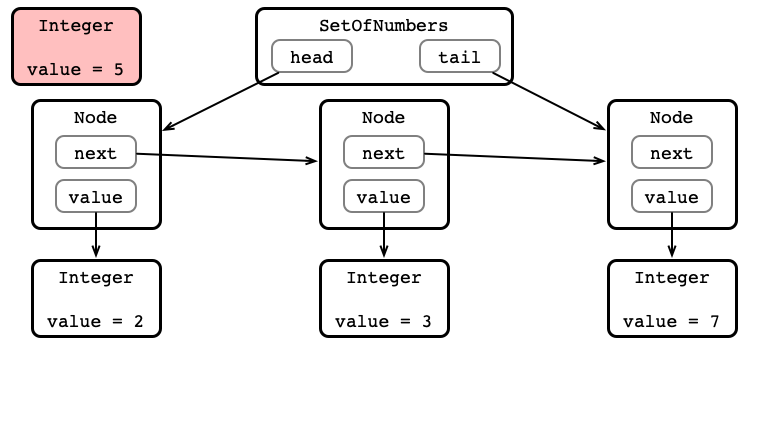Idea

Use procedure similar to remove method:

• store prev and curr nodes
• iterate over list until
1. curr value is at least 5
2. prev value is smaller than 5
• insert node storing value 5 between prev and curr

Insertion in PicturesInsertion in Pictures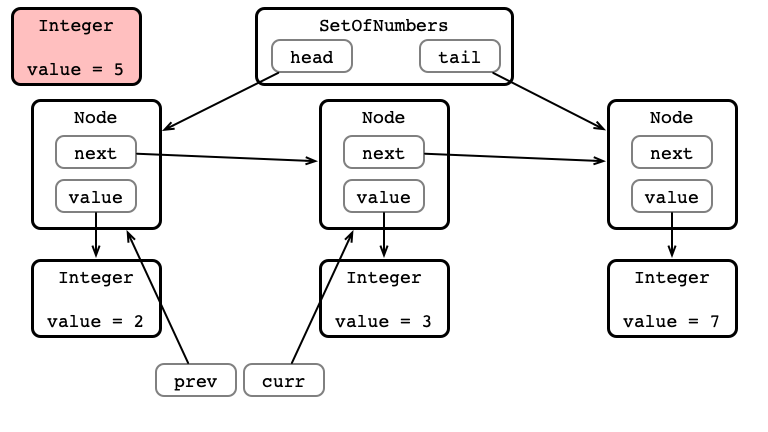Insertion in Pictures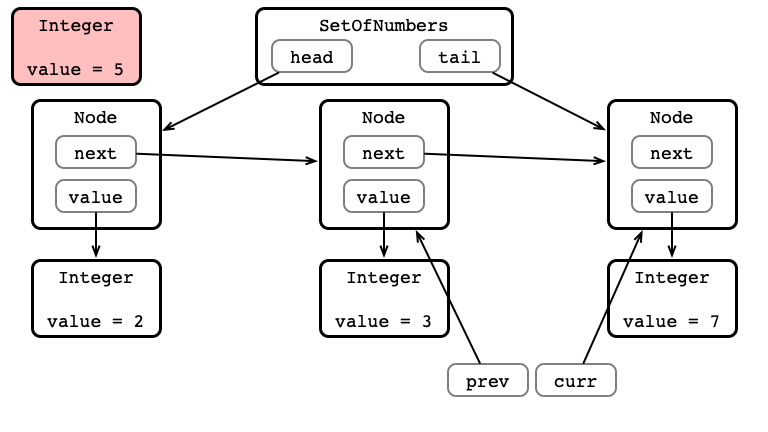Insertion in Pictures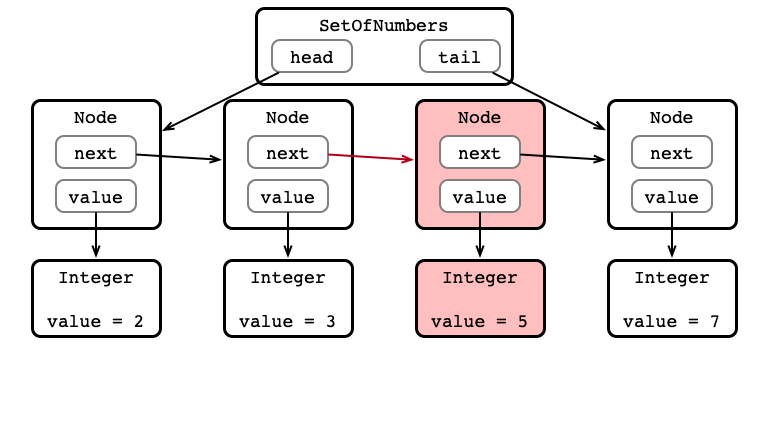Insertion in Code

Special cases:

1. inserted value is smallest
2. inserted value is largest
• update tail

Insertion in Code

public void insert (T item) {

Node nd = new Node(item);

// check if empty
if (tail == null) {
head = nd; tail = nd; iter = nd;
return;
}

// check if insert at head
return;
}

Node curr = prev.next;

// find location to insert item: prev.item < item <= curr.item
while (curr != null && item > curr.item) {
prev = curr;
curr = curr.next;
}

// check if tail insert
if (curr == null) {
prev.next = nd; tail = nd;
return;
}

prev.next = nd;
nd.next = curr;
}

What is the Problem?

public void insert (T item) {

Node nd = new Node(item);

if (tail == null) {
head = nd; tail = nd; iter = nd;
return;
}

return;
}

Node curr = prev.next;

while (curr != null && item > curr.item) {
prev = curr;
curr = curr.next;
}

if (curr == null) {
prev.next = nd; tail = nd;
return;
}

prev.next = nd;
nd.next = curr;
}

Requirement

In order to store a sorted collection, must be able to compare pairs of elements.

• compare numbers by value
• compare Strings alphabetically
• compare Animals by…
• …species?
• …name?
• …age?

Limitation

It doesn’t make sense to compare everything!

Goals:

1. Specify that a particular type of object does support comparisons
• need means of signalling that a class supports comparison
2. Require that only objects that can be compared are stored in a SortedList
• otherwise, sorting doesn’t make sense

Interfaces

What is an Interface?

A way of specifying what a class can/must do

• specify functionality
• does not specify implementation
• a class providing required functionality implements the interface
• may be many possible ways to implement an interface

The Comparable Interface

Java defines the Comparable<T> interface:

For a class to be Comparable it must have a method

• public int compareTo(T o)

Comparable Semantics

If a and b are Comparable objects:

• if a.compareTo(b) is less than 0, then a is “smaller than” b
• if a.compareTo(b) is greater than 0, then a is “larger than” b
• if a.compareTo(b) is 0 then they are treated as equal

Classes Implementing Comparable

• Integer, Double, …
• compare numerical values as you’d expect
• String
• compare alphabetically

Making Animals Comparable

To implement an interface, must:

1. Declare that class implements the interface:
public class Animal implements Comparable<Animal> {
...
}

2. Implement the methods required by the interface
public class Animal implements Comparable<Animal> {
...
public int compareTo(Animal a) { ... }
}

A Question

How should we compare two Animals?

Back to our Sorted List

Create a new class SortedList

• stores elements as in our GenericList
• elements in sorted order
• elements must implement Comparable

A new declaration:

// T a generic type, but must implement the Comparable<T>
// interface

public class SortedList<T extends Comparable<T>> {
...
}

Why Interfaces?

An interface is a contract:

1. In order to implement the interface, you must provide certain functionality
2. If you provide that functionality, I can write code that uses the functionality to perform some task
• I don’t need to know how you provide functionality

Sorting example:

• We wrote code to store a sorted list of items
• Works for any class implementing Comparable
• The class determines what is meant by comparison

Good Design

Separate interface from implementation

• Better encapsulation
• Explicit functionality of the class
• Explicit requirements to use existing functionality
• Can provide different implementations of same interface
• sort by species/name? sort by age? by height?
• different implementations might be appropriate for different contexts